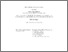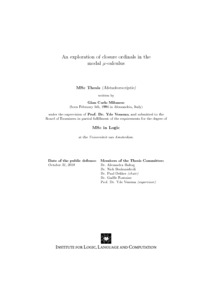# MoL-2018-32: An exploration of closure ordinals in the modal μ-calculus

MoL-2018-32: Milanese, Gian Carlo (2018) An exploration of closure ordinals in the modal μ-calculus. [Pre-print]Preview Text MoL-2018-32.text.pdf Download (2MB) | Preview

## Abstract

In this thesis we explore closure ordinals of the modal μ-calculus. The closure ordinal of a μ-calculus formula φ(x) is the least ordinal α, if it exists, such that the iteration of the meaning function φ^S_x starting from the emptyset converges to its least fixed point in at most α many steps on every model S. Our goal is to write an accessible introduction to this field by recalling some fundamental recent results and contributing with a few of our own. We provide a syntactic characterisation of the fragment of the μ-calculus corresponding to the semantic property of normality in a finite set of variables (which coincides with the property of having closure ordinal 0), we provide a syntactic characterisation of the fragment of the μ-calculus corresponding to the property of continuity on finitely branching models, we construct a formula φ_n with closure ordinal ω^n on bidirectional models for an arbitrary n∈ω, we construct a formula φ_α with closure ordinal α on bidirectional models for an arbitrary α < ω^ω , and we prove that the set of closure ordinals on bidirectional models is closed under ordinal sum (as an adaptation to this setting of the original result by Gouveia and Santocanale).

Item Type: Pre-print MoL-2018-32 Master of Logic Thesis (MoL) Series 2018 LogicMathematics Dr Marco Vervoort 29 Nov 2018 15:51 29 Nov 2018 15:51 https://eprints.illc.uva.nl/id/eprint/1648View Item